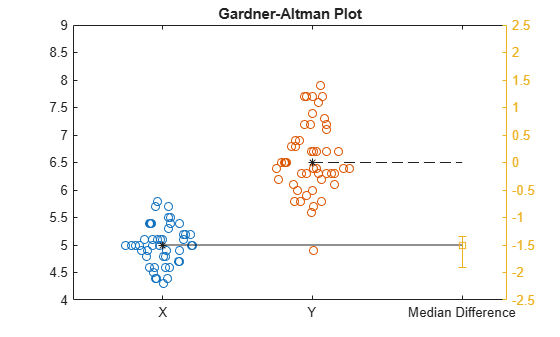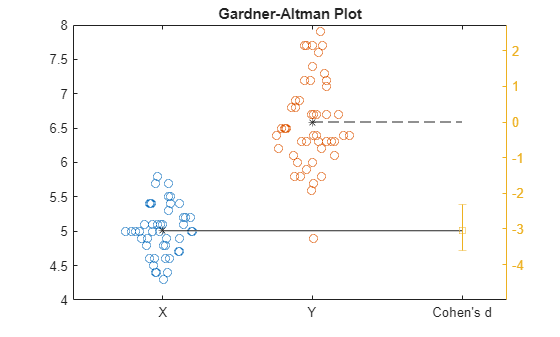# meanEffectSize

One-sample or two-sample effect size computations

## Syntax

``Effect = meanEffectSize(X)``
``Effect = meanEffectSize(X,Y)``
``Effect = meanEffectSize(X,Y,Name=Value)``

## Description

example

````Effect = meanEffectSize(X)` computes the mean difference effect size for a single sample `X` against the default mean value of 0.```

example

````Effect = meanEffectSize(X,Y)` computes the mean difference effect size for two samples `X` and `Y`.```

example

````Effect = meanEffectSize(X,Y,Name=Value)` computes the mean difference effect size for options specified using one or more of the `Name=Value` arguments.```

## Examples

collapse all

Load the stock returns data and define the variable for which to compare the mean effect size.

```load stockreturns x = stocks(:,1);```

Compute the mean difference effect size of the stock returns compared to the default mean value of 0 and compute the 95% confidence intervals for the effect size.

`effect = meanEffectSize(x)`
```effect=1×2 table Effect ConfidenceIntervals ________ ______________________ MeanDifference -0.20597 -0.41283 0.00087954 ```

`meanEffectSize` uses the exact method to estimate the confidence intervals when you use mean difference effect size.

You can also specify the mean value you want to compare against.

`effect = meanEffectSize(x,Mean=-1)`
```effect=1×2 table Effect ConfidenceIntervals _______ ___________________ MeanDifference 0.79403 0.58717 1.0009 ```

Load Fisher's iris data and define the variables for which to compare.

```load fisheriris species2 = categorical(species); x = meas(species2=='setosa'); y = meas(species2=='virginica');```

Compute the median difference effect size of the observations from two independent samples.

`effect = meanEffectSize(x,y,Effect="mediandiff")`
```effect=1×2 table Effect ConfidenceIntervals ______ ___________________ MedianDifference -1.5 -1.8259 -1.3 ```

`meanEffectSize` by default assumes independent samples (that is, `Paired=false`). The function uses bootstrapping to estimate the confidence intervals when effect type is median difference.

Visualize the median difference effect size using Gardner-Altman plot.

`gardnerAltmanPlot(x,y,Effect="mediandiff");`Gardner-Altman plot displays the two sample data on the left. The median of the sample `Y` corresponds to the zero effect size on the effect size axis, which is the yellow axis line on the right. The median of the sample `X` corresponds to the value of the effect size on the effect size axis. The plot displays the actual median difference effect size value and the confidence intervals with the vertical error bar.

Load Fisher's iris data and define the variables for which to compare.

```load fisheriris species2 = categorical(species); x = meas(species2=='setosa'); y = meas(species2=='virginica');```

Compute the Cohen's d effect size for the observations from two independent samples and compute the 95% confidence intervals for the effect size. `meanEffectSize` by default uses the exact formula based on the noncentral t-distribution to estimate the confidence intervals when the effect type is Cohen's d. Specify the bootstrapping options:

• Tell `meanEffectSize` to use bootstrapping for confidence interval computation.

• Use parallel computing for bootstrapping computations. You need Parallel Computing Toolbox™ for this option.

• Use 3000 bootstrap replicas.

```rng(123) % For reproducibility effect = meanEffectSize(x,y,Effect="cohen",ConfidenceIntervalType="bootstrap", ... BootstrapOptions=statset(UseParallel=true),NumBootstraps=3000)```
```Starting parallel pool (parpool) using the 'local' profile ... Connected to the parallel pool (number of workers: 6). ```
```effect=1×2 table Effect ConfidenceIntervals _______ ___________________ CohensD -3.0536 -3.5611 -2.3219 ```

Use the same options in Gardner-Altman plot to visualize the effect size.

```gardnerAltmanPlot(x,y,Effect="cohen",ConfidenceIntervalType="bootstrap", ... BootstrapOptions=statset(UseParallel=true),NumBootstraps=3000);```Gardner-Altman plot displays the two sample data on the left. The mean of the sample `Y` corresponds to the zero effect size on the effect size axis, which is the yellow axis line on the right. The mean of the sample `X` corresponds to the value of the effect size on the effect size axis. The plot displays the Cohen's d effect size value and the confidence intervals with the vertical error bar.

Load exam grades data and define the variables for which to compare.

```load examgrades x = grades(:,1); y = grades(:,2);```

Compute the mean difference effect size of the grades from the paired samples and 95% confidence intervals for the effect size.

`effect = meanEffectSize(x,y,Paired=true)`
```effect=1×2 table Effect ConfidenceIntervals ________ ___________________ MeanDifference 0.016667 -1.3311 1.3644 ```

`meanEffectSize` uses the exact method to estimate the confidence intervals when you use mean difference effect size.

You can use a different effect size type (note that you can't use Glass's delta for paired samples). Use robust Cohen's d to compare the paired-sample means. Compute the 97% confidence intervals for the effect size.

`effect = meanEffectSize(x,y,Paired=true,Effect="robustcohen",Alpha=0.03)`
```effect=1×2 table Effect ConfidenceIntervals ________ ___________________ RobustCohensD 0.059128 -0.1405 0.26573 ```

`meanEffectSize` uses bootstrapping to estimate the confidence intervals when you use robust Cohen's d as the effect size.

Visualize the effect size using Gardner-Altman plot. Again use robust Cohen's d as the effect size and compute the 97% confidence intervals.

`gardnerAltmanPlot(x,y,Paired=true,Effect="robustcohen",Alpha=0.03);`Gardner-Altman plot displays the paired data on the left. Blue lines show the values that are increasing and the red lines show the values that are decreasing from the first sample to the corresponding values in the paired sample, respectively. On the right side of the plot, you see the robust Cohen's d effect size with the 97% confidence intervals.

## Input Arguments

collapse all

Input data, specified as a numeric vector.

Data Types: `single` | `double`

Input data, specified as a numeric vector.

Data Types: `single` | `double`

### Name-Value Arguments

Specify optional pairs of arguments as `Name1=Value1,...,NameN=ValueN`, where `Name` is the argument name and `Value` is the corresponding value. Name-value arguments must appear after other arguments, but the order of the pairs does not matter.

Example: `Effect="cliff",Alpha=0.03,ConfidenceIntervalType="bootstrap",VarianceType=unequal` specifies to use the Cliff's Delta effect size, compute the 97% confidence intervals using bootstrapping, and assume the samples come from populations with unequal variances.

Confidence level, specified as a numeric value from 0 to 1. Default value of `Alpha`, 0.05, corresponds to 95% confidence level.

Example: `Alpha=0.025`

Data Types: `single` | `double`

Options for bootstrap confidence interval computation in parallel, specified as a structure generated by using `statset('bootci')`. `meanEffectSize` uses the following fields:

FieldDescription
`'Streams'`

A `RandStream` object or cell array of such objects. If you do not specify `Streams`, `meanEffectSize` uses the default stream or streams. If you specify `Streams`, use a single object except when all of the following conditions exist:

• You have an open parallel pool.

• `UseParallel` is `true`.

• `UseSubstreams` is `false`.

In this case, use a cell array the same size as the parallel pool. If a parallel pool is not open, then `Streams` must supply a single random number stream.

`'UseParallel'`The default is `false`, indicating serial computation.
`'UseSubstreams'`Set to `true` to compute in parallel in a reproducible fashion. The default is `false`. To compute reproducibly, set `Streams` to a type allowing substreams: `'mlfg6331_64'` or `'mrg32k3a'`.

Computing bootstrap confidence intervals in parallel requires Parallel Computing Toolbox™.

Example: `BootstrapOptions=options`

Data Types: `struct`

Type of confidence interval to compute, specified as `"exact"`, `"bootstrap"`, or `"none"`. The default is `"exact"` when there is an exact formula for the effect size or `"bootstrap"` otherwise. `"none"` is for not computing any confidence intervals.

Default is `"exact"` for Cliff's Delta, Glass's delta, mean difference, and Cohen's d and `"bootstrap"` for Kolmogorov-Smirnov statistic, median difference, and Robust Cohen's d. If you specify confidence interval type as `"exact"` for Kolmogorov-Smirnov statistic, median difference, and Robust Cohen's d, `meanEffectSize` returns an error.

Example: `ConfidenceIntervalType="none"`

Data Types: `string` | `char`

Effect size type to compute, specified as one of or a cell array of the following built-in options.

Options for single-sample input

Effect size optionDefinition
`"cohen"`Cohen's d for single-sample input.
`"meandiff"`Mean difference.
`"robustcohen"`Robust Cohen's d for single-sample input.

Options for two-sample input

Effect size optionDefinition
`"cohen"`Cohen's d for two-sample input.
`"cliff"`Cliff's Delta.
`"glass"`

Glass's delta. `meanEffectSize` doesn't support this option for paired data.

`"kstest"`Kolmogorov-Smirnov statistic.
`"mediandiff"`Median difference.
`"meandiff"`Mean difference.
`"robustcohen"`Robust Cohen's d for two-sample input.

Example: `Effect="glass"`

Data Types: `string` | `char` | `cell`

Known population mean value to compare against, specified as a scalar value. This option is only for single-sample data.

Example: `Mean=10`

Data Types: `single` | `double`

Number of bootstrap replicas to use when computing the bootstrap confidence intervals, specified as a positive integer.

Example: `NumBootstraps=1500`

Data Types: `single` | `double`

Indicator for paired samples, specified as a logical value.

Example: `Paired="true"`

Data Types: `logical`

Population variance assumption for two samples, specified as either `"equal"` or `"unequal"`. If `Paired` is `"true"`, then `VarianceType` must be `"equal"`.

Example: `VarianceType="unequal"`

Data Types: `string` | `char`

## Output Arguments

collapse all

Effect size information, returned as a table. `Effect` has a row for each effect size computed and a column for the value of the effect size, and a column for the confidence intervals for that effect size, if they are computed.

Data Types: `table`

## Algorithms

collapse all

### Effect Sizes

• Cliff's Delta

• Unpaired data

`$\delta =\frac{\sum _{i,j=1}^{{n}_{1},{n}_{2}}\left({x}_{i}>{y}_{j}\right)-\left({x}_{i}<{y}_{j}\right)}{{n}_{1}*{n}_{2}},$`

where n1 is the size of the first sample and n2 is the size of the second sample.

• Paired data

`meanEffectSize` uses the between-group delta, which is comparing the differences from `x` and `y`, but excluding comparisons of paired data. For n paired samples, this results in n(n-1) comparisons .

• Cohen's d

`meanEffectSize` computes the unbiased estimate of Cohen's d, which is also known as Hedge's g.

• One-sample

`$d=J\left(df\right)*\frac{\left(\overline{x}-\mu \right)}{s}$`
• Two-sample

`$d=J\left(df\right)*\frac{\left(\overline{x}-\overline{y}\right)}{s}$`

where df is the degrees of freedom, μ is the known population mean to compare against, s is the pooled standard deviation, and J(df) is the bias correction term. The pooled standard deviation and the bias correction term are defined as follows, respectively.

`$s=\sqrt{\frac{\left({n}_{1}-1\right){s}_{1}^{2}+\left({n}_{2}-1\right){s}_{2}^{2}}{{n}_{1}+{n}_{2}-2}},$`

where n1 is the size of the first sample and n2 is the size of the second sample.

`$J\left(df\right)=\frac{\Gamma \left(df/2\right)}{\sqrt{df/2}\Gamma \left(\left(df-1\right)/2\right)},$`

where $\Gamma \left(\right)$ is the gamma function.

Cohen's d follows a noncentral t-distribution, and uses that to derive the confidence intervals. Hence, `meanEffectSize` by default uses `"exact"` to compute the confidence intervals for the effect size. See  and  to see the derivation of the confidence intervals for paired versus unpaired input data.

• Glass's Delta

`$D=\frac{\overline{x}-\overline{y}}{{s}_{x}},$`

where sx is the standard deviation of the control group. `meanEffectSize` uses the data in `x` as the control group. If you wish to use the other sample as the control group, you can swap the data in `x` and `y` and swap the sign of the test result.

Similar to Cohen's d, Glass's delta also follows a noncentral t-distribution, and uses that to derive the confidence intervals. Hence, `meanEffectSize` by default uses `"exact"` to compute the confidence intervals for the effect size . You can't use this effect size for paired samples.

• Kolmogorov-Smirnov Test Statistic

This two-sample test statistic is the same as given in Two-Sample Kolmogorov-Smirnov Test. `meanEffectSize` uses bootstrapping to compute the confidence intervals.

• Mean Difference

• One-sample

`$m=\left(\overline{x}-\mu \right)$`
• Two-sample

`$m=\left(\overline{x}-\overline{y}\right)$`

`meanEffectSize` computes the confidence intervals using the t-distribution (using pooled standard deviation in the two-sample case. In case of unequal variance assumption for two samples, the confidence intervals are called Welch-Satterthwaite confidence intervals). The function by default uses the `"exact"` method to compute the confidence intervals.

• Median Difference

`meanEffectSize` computes the confidence intervals using bootstrapping for this effect size.

• Robust Cohen's d

`$d=0.643*J\left(df\right)*\frac{\left({\overline{x}}_{t}-{\overline{y}}_{t}\right)}{{s}_{w}},$`

where ${\overline{x}}_{t}$ and ${\overline{y}}_{t}$ are the 20% trimmed mean of data in` x` and `y`, respectively. ${\text{s}}_{w}$ is the pooled 20% Winsorized variance .

`meanEffectSize` computes the confidence intervals using bootstrapping for this effect size.

 Cousineau, Denis and Jean-Christophe Goulet-Pelletier. "A study of confidence intervals for Cohen's d in within-subject designs with new proposals". The Quantitative Methods for Psychology, 2021.

 Algina, James, H. J. Keselman, and R. D. Penfield. "An Alternative to Cohen's Standardized Mean Difference Effect Size: A Robust Parameter and Confidence Interval in the Two Independent Groups Case". Psychological Methods, Vol. 10, No. 3, pp. 317–328. 2005.

 Hess, Melinda and Jeffrey Kromrey. "Robust Confidence Intervals for Effect Sizes: A Comparative Study of Cohen's d and Cliff's Delta Under Non-normality and Heterogeneous Variances". Annual Meeting of the American Educational Research Association. 2004.

 Delacre, Marie, Daniel Lakens, Christophe Ley, Limin Liu, and Christophe Leys. "Why Hedges G's Based on the Non-pooled Standard Deviation Should Be Reported with Welch's T-test". 2021.

 Gardner, M. J. and D. G. Altman. "Confidence intervals rather than P values: estimation rather than hypothesis testing". BMJ, Vol. 292 No. 6522, pp. 746–750. 1986.